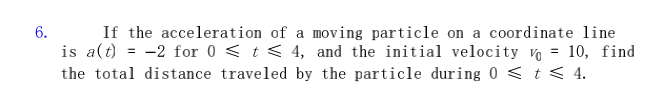### ¿Todavía tienes preguntas de matemáticas?

Pregunte a nuestros tutores expertos
Algebra
Pregunta6. If the acceleration of a moving particle on a coordinate line is $$a ( t ) = - 2$$ for $$0 \leq t \leq 4$$ , and the initial velocity $$v _ { 0 } = 10$$ , find the total distance traveled by the particle during $$0 \leq t \leq 4$$ .